### Discontinuous Opening

The discontinuous opening is the downstream element of the boundary element pair defining a change in channel slope. The following constants have to be specified on the line beneath the *FLUID SECTION,TYPE=CHANNEL DISCONTINUOUS OPENING card:

• the width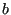•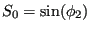(Figure 122; if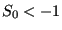the slope is calculated from the coordinates of the end nodes belonging to the element)
• the length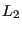(Figure 122; if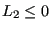the length is calculated from the coordinates of the end nodes belonging to the element)
• the trapezoid angle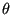• the grain diameter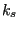for the White-Colebrook law or the Manning constant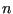for the Manning law (in the latter case the user has to specify the parameter MANNING on the *FLUID SECTION card)
• the number of the upstream discontinuous slope element.

The lengthis typically small compared to the length of the adjacent channel branches.

Example files: channel6.